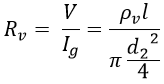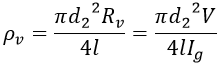# Direct Deflection Method - Measuring High Resistance, Surface & Volume Resistivity

#### The different methods employed for measurement of high resistance are,Loss of charge methodDirect deflection methodBy using Megger.

In the last article, we have seen measurement high resistance using the loss of charge method. In this let us see the measuring of high resistance using the direct deflection method.

## Direct Deflection Method :

In this method the high resistance under measurement is determined by the deflection of the galvanometer, hence the method called Direct Deflection Method. This method is similar to the principle of the ammeter-voltmeter method used for measuring low and medium resistance. It is mainly used for measuring the insulation resistance of a cable and insulating materials.

The below shows the measurement of high insulation resistance (more than 1000Ω). The method is similar to the ammeter-voltmeter method but here the microammeter is replaced by a very high sensitive galvanometer and connected in series with the resistance to be measured.

When the supply is given, the galvanometer shows the deflection proportional to the current between core and metal sheath. Here the guard rings or guard wire eliminates the leakage currents over the surface of the insulation. Hence, the insulation resistance between the core and metal sheath is determined by the voltage, and the current flowing through the galvanometer.

A typical direct deflection method for measuring the insulation resistance of a cable without a metal sheath is shown below. Here, before the measurement process, the cable is immersed in water contained in a tank maintained at constant temperature for 24 hrs. These account for any defects in the insulation of the cable by soaking the water through it.

The negative terminal of the battery is connected to the tank, which causes the leakage currents to pass to the battery rather than to the galvanometer without affecting its deflection. Whereas the positive terminal is connected at the end of the cable by twisting the wire around the insulation.

At starting before supplying the voltage, the galvanometer should be shortened by the switch, to protect from the sudden inrush charging currents due to the capacitance of the cable. The galvanometer is then connected in the circuit after the application of voltage. A high resistance shunt is connected to the circuit for the protection of the galvanometer and also to provide multiple ranges.

The galvanometer measures the current between the cable core and the insulation sheath. The insulation resistance is then determined, the true value of insulation resistance is given by subtracting the shunt resistance from the determined resistance.

The guard wire is incorporated to bypass the leakage currents IL flowing through the galvanometer so that its reading doesn't get affected. The voltage applied should be maintained constant and the galvanometer should be highly sensitive with high resistance and critically damped.

## Measurement of Surface and Volume Resistivity :

The direct deflection method can also be used to determine the insulation resistance of materials available in sheet form by measuring the surface resistivity and volume resistivity of the material sample.

The specimen of the insulating material is attached with two electrodes on the surface, the upper and lower electrodes. A small electrode is placed on the edge of the upper electrode that acts as a guard ring to eliminate the flow of leakage currents through the galvanometer.

Hence the leakage currents flow between the lower electrode and guard electrode without affecting the galvanometer deflection. The schematic diagrams for measuring the surface and volume resistivity of a sample are shown below.

### Surface Resistivity :

The surface resistivity is the resistance between opposite edges of a square area of the surface of the material. It varies with variation in the weather conditions around the test sample i.e., weather conditions on the surface and humidity.

The surface resistivity is measured by connecting the galvanometer between the guard electrode and the lower electrode as shown in figure (a) above. Thus galvanometer shows the deflection proportional to leakage current.

### Volume Resistivity :

The specific resistance of the material or electrical resistivity is said to be known as volume resistivity. In order to measure volume resistivity, the galvanometer is connected in series with the upper electrode as shown in figure (b) above.

#### Let,d2 = Diameter of the upper electrodel = Length of surface path or thicknessV = Voltage appliedIg = Current through the galvanometer. The resistance of the specimen is,From the above equation, the volume resistivity can be expressed as,Do not enter any spam links and messages